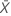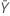# Methods and formulas for Test mean / reference mean for Equivalence Test with Paired Data

The following methods and formulas are used for testing the ratio between the test mean and the reference mean.

## Ratio

### Notation

TermDescription
ρRatioTest meanReference mean

## Equivalence limits

Let k1 be the value that you specify for the lower limit and k2 be the value that you specify for the upper limit. By default, the lower equivalence limit, δ1, is given by:and the upper equivalence limit, δ2, is given by:## Degrees of freedom (DF)

### Notation

TermDescription
vDegrees of freedom
nNumber of pairs of observations

## S12

S12 represents the sample covariance between the X values and the Y values. This value is used in the calculations for the CI and T-values.

### Notation

TermDescription
XiThe ith observation in the test sample, such that (Xi, Yi) is the ith pair of observations
YiThe ith observation in the reference sample, such that ( Xi, Yi) is the ith pair of observationsMean of the test sampleMean of the reference sample
nNumber of pairs of observations

## Confidence interval

Minitab cannot calculate the confidence interval (CI) if either of the following two conditions are satisfied:

If the conditions are satisfied, Minitab calculates the CI based on the method used for the analysis.
• 100(1 - α)% CI

By default, Minitab calculates the 100(1 - α)% CI for ρ as follows:

CI = [min(C, ρL), max(C, ρU)]

where:
• 100(1 - 2α)% CI

If you select the option to use the 100(1 - 2α)% CI, then the CI is given by the following:

CI = [ρL, ρU]
• One-sided intervals

For a hypothesis of Test mean / reference mean > lower limit, the 100(1 - α)% lower bound is equal to ρL.

For a hypothesis of Test mean / reference mean < upper limit, the 100(1 - α)% upper bound is equal to ρU.

### Notation

TermDescriptionMean of the test sampleMean of the reference sample
S12Sample covariance between the X values and the Y values
S1Standard deviation of the test sample
nthe sample size
S2Standard deviation of the reference sample
δ1Lower equivalence limit
δ2Upper equivalence limit
vDegrees of freedom
αSignificance level for the test (alpha)
t1-α,vUpper 1 - α critical value for a t-distribution with v degrees of freedom

## T-values

Let t1 be the t-value for the hypothesis,, and let t2 be the t-value for the hypothesis,, whereis the ratio of the mean of the test population to the mean of the reference population.

### Notation

TermDescriptionMean of the test sampleMean of the reference sample
S1Standard deviation of the test sample
S2Standard deviation of the reference sample
S12Correlation between the X values and the Y values
nNumber of pairs of observations
δ1Lower equivalence limit
δ2Upper equivalence limit
ΛUnknown ratio of the mean of the test population to the mean of the reference population

## P-values

The probability, PH0, for each null hypothesis is given by the following:

If, then:

H0 P-Value### Notation

TermDescription
ΛUnknown ratio of the mean of the test population to the mean of the reference population
δ1Lower equivalence limit
δ2Upper equivalence limit
vDegrees of freedom
Tt-distribution with v degrees of freedom
t1 t-value for the hypothesist2 t-value for the hypothesis###### Note

For information on how the t-values are calculated, see the section on t-values.

By using this site you agree to the use of cookies for analytics and personalized content.  Read our policy